# Tamil Nadu 12th Computer Science Model Question Paper 3 English Medium

Students can Download Tamil Nadu 12th Computer Science Model Question Paper 3 English Medium Pdf, Tamil Nadu 12th Computer Science Model Question Papers helps you to revise the complete Tamilnadu State Board New Syllabus and score more marks in your examinations.

## TN State Board 12th Computer Science Model Question Paper 3 English Medium

General Instructions:

1. The question paper comprises of four parts.
2. You are to attempt all the parts. An internal choice of questions is provided wherever applicable.
3. All questions of Part I, II, III and IV are to be attempted separately.
4. Question numbers 1 to 15 in Part I are Multiple Choice Questions of one mark each.
These are to be answered by choosing the most suitable answer from the given four alternatives and writing the option code and the corresponding answer
5. Question numbers 16 to 24 in Part II are two-mark questions. These are to be answered in about one or two sentences.
6. Question numbers 25 to 33 in Part III are three-mark questions. These are to be answered in about three to five short sentences.
7. Question numbers 34 to 38 in Part IV are five-mark questions. These are to be answered in detail Draw diagrams wherever necessary.

Time: 3 Hours
Maximum Marks: 70

PART – I

Choose the correct answer. Answer all the questions [15 x 1 = 15]

Question 1.
………. function returns the smallest integer greater than or equal to x.
(a) sqrt
(b) flow
(c) floor
(d) ceil
(d) ceil

Question 2.
……….. is used to indicate blocks of code in python.
(a) Spaces
(b) {}
(c) []
(d) <>
(a) SpacesQuestion 3.
Which amongst this is not a Jump Statement?
(a) for
(b) goto
(c) continue
(d) break
(a) for

Question 4.
How many formats are there for format ()?
(a) 12
(b) 5
(c) 3
(d) 1
(c) 3

Question 5.
Stings in python can be created using…………
{a) ” ”
(b) ’ ’
(c) ”’ ”’
(d) all of these
(d) all of these

Question 6.
……….. function is used to generate a series of values in python.
(a) range
(b) series
(c) fill series
(d) auto fill
(a) range

Question 7.
Duplicate row is. removed in………..
(a) α
(b) π
(c) x
(d) σ
(b) π

Question 8.
Grant and Revoke commands comes under…………
(a) DML
(b) DCL
(c) DQL
(d) DDL
(b) DCL

Question 9.
A CSV file is also called as………..file.
(a) flat
(b) 3D
(c) string
(d) random
(a) flat

Question 10.
The module which allows you to interface with the Windows OS is…………
(a) OS module
(b) SYS module
(c) CSV module
(d) getopt module
(a) OS module

Question 11.
The most commonly used command in SQL is…………
(a) cursor
(b) select
(c) execute
(d) commit
(b) select

Question 12.
Which command is used to accept data during runtime in python?
(a) Insert ()
(b) Input ()
(c) create
(d) update ()
(b) Input ()

Question 13.
The representation of information in a graphic format is…………
(a) chart
(b) graphics
(c) infographics
(d) graphs
(c) infographics

Question 14.
What does name contains?
(a) clt filename
(b) main () name
(c) python filename
(d) OS module name
(c) python filenameQuestion 15.
If the precision exceeds 64, then it is………….
(a) numeric
(b) real
(c) float
(d) decimal
(c) float

PART – II

Answer any six questions. Question No. 21 is compulsory. [6 × 2 = 12]

Question 16.
Define Pseudo code.

1. Pseudo code is a mix of programming-language-like constructs and Plain English.
2. Pseudo code is a notation similar to programming languages.
3. Algorithms expressed in pseudo code are not intended to be executed by computers, but for communication among people.

Question 17.
What is searching? Write its types.
A search algorithm is the step-by-step procedure used to locate specific data among a collection of data. Types of searching algorithms are

1. Linear search
2. Binary search
3. Hash search
4. Binary Tree search

Question 18.
Name the factors where the program execution time depends on.
The program execution time depends on:

1. Speed of the machine
2. Compiler and other system Software tools
3. Operating System
4. Programming language used
5. Volume of data required

Question 19.
Give any 6 keywords in python.
false, class, none, continue, finally, return, is

Question 20.
Give the syntax of while loop.
The syntax of while loop in Python has the following syntax:
Syntax:
while:
statements block 1 [else:
statements block2]

Question 21.
Write a program to display all 3 digit odd numbers.
Odd Number (3 digits)
for a in range (100, 1000):
if a % 2 = 1:
print b
Output:
101, 103, 105,107 …… 997, 999

Question 22.
Write note on pass statement.
pass statement in Python programming is a null statement, pass statement when executed by the interpreter it is completely ignored. Nothing happens when pass is executed, it results in no operation. pass statement can be used in ‘if’ clause as well as within loop construct, when you do not want any statements or commands within that block to be executed.

Question 23.
Define default arguments.
In Python the default argument is an argument that takes a default value if no value is provided in the function call. The following example uses default arguments, that prints default salary when no argument is passed, def printinfo (sal = 3500):Question 24.
Write a python program to find the length of the string.
str = input(“Enter a string: “) print (len(str))
Output:
Enter a string: HELLO
5

PART – III

Answer any six questions. Question No. 29 is compulsory. [6 x 3 = 18]

Question 25.
Differentiate List and Dictionary.

1. List is an ordered set of elements. But, a dictionary is a data structure that is used for matching one element (Key) with another (Value).
2. The index values can be used to access a particular element. But, in dictionary key represents index. Remember that, key may be a number of a string.
3. Lists are used to look up a value whereas a dictionary is used to take one value and look up another value.

Question 26.
What is normalization?

1. Normalization is a process of organizing the data in the database to avoid data redundancy and to improve data integrity.
2. Database normalization was first proposed by Dr. Edgar F Codd as an integral part of RDBMS.
These rules are known as E F Codd Rules.

Question 27.
What are the advantages of DBMS?

1. Segregation of application program
2. Minimal data duplication or Data Redundancy
3. Easy retrieval of data using the Query Language
4. Reduced development time and maintenance

Question 28.
Write the use of savepoint command with an example.
SAVEPOINT command
The SAVEPOINT command is used to temporarily save a transaction so that you can rollback to the point whenever required. The different states of our table can be saved at anytime using different names and the rollback to that state can be done using the ROLLBACK command.
SAVEPOINT savepoint_name;
UPDATE Student SET Name = ‘Mini ’ WHERE Admno = 105;
SAVEPOINT A;

Question 29.
Give a program for CSV file with a line terminator.
import csv
Data = [[‘Fruit’, ‘Quantity’], [‘Apple’, ‘5’], [‘Banana’, ‘7’], [‘Mango’, ‘8’]]
csv.register_dialect(‘myDialect’, delimiter ‘|’, lineterminator = ‘\n’)
with open(‘c:\\pyprg\ \chl3\\line.csv’, ‘w’) as f:
writer = csv.writer(f, dialect = ‘myDialect’)
writer.writerows(Data)
f.close()
Output

 Fruit Quantity Apple 5 Banana 7 Mango 8

Question 30.
What is MinGW?
(MinGW refers to a set of runtime header files, used in compiling and linking the code of C, C++ and FORTRAN to be run on Windows Operating System.

MinGw-W64 (versionofMinGW) is the best compiler for C++ on Windows. To compile and execute the C++ program, you need ‘g++’ for Windows. MinGW allows to compile and execute C++ program dynamically through Python program using g++.

Python program that contains the C++ coding can be executed only through minGW-w64 project run terminal. The run terminal open the command-line window through which Python program should be executed.Question 31.
How will you import modules in python?
We can import the definitions inside a module to another module. We use the import keyword . to do this. To import the module factorial we type the following in the Python prompt.
>> import factorial
Using the module name we can access the functions defined inside the module. The dot(.) operator is used to access the functions. The syntax for accessing the functions from the module is
<module name><function name>
For example: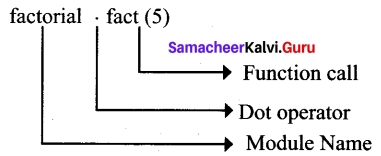>>> factorial.fact(5)
120

Question 32.
Write a command to populate record in a table.
To populate (add record) the table “INSERT” command is passed to SQLite. “execute” method executes the SQL command to perform some action. In most cases, you will not literally insert data into a SQL table. You will rather have a lot of data inside of some Python data type e.g. a dictionary or a list, which has to be used as the input of the insert statement.

Question 33.
Design an algorithm to find square of the given number and display the result.
Problem: Design an algorithm to find square of the given number and display the result. The algorithm can be written as:
Step 1 – start the process
Step 2 – get the input x
Step 3 – calculate the square by multiplying the input value ie., square ← x * x
Step 4 – display the result square
Step 5 – stop
Algorithm could be designed to get a solution of a given problem. A problem can be solved in many ways. Among many algorithms the optimistic one can be taken for implementation.

PART – IV

Answer all the questions. [5 × 5 = 25]

Question 34 (a).
Explain about any 3 string operators in python with suitable example.
String Operators
Python provides the following operators for string operations. These operators are useful to manipulate string.
(i) Concatenation (+)
Joining of two or more strings is called as Concatenation. The plus (+) operator is used to concatenate strings in python.
Example
>>> “welcome” + “Python”
‘welcomePython’

(ii) Append (+ =)
Adding more strings at the end of an existing string is known as append. The operator += is used to append a new string with an existing string.
Example .
>>> strl =” Welcome to ”
>>> strl+=”Leam Python”
>>> print (strl)
Welcome to Learn Python

(iii) Repeating (*)
The multiplication operator (*) is used to display a string in multiple number of times.
Example
>>> strl = “Welcome ”
>>> print (strl *4)
Welcome Welcome Welcome Welcome

(iv) String slicing
Slice is a substring of a main string. A substring can be taken from the original string by using [ ] operator and index or subscript values. Thus, [ ] is also known as slicing operator. Using slice operator, you have to slice one or more substrings from a main string.
General format of slice operation:
str [start:end]
Where start is the beginning index and end is the last index value of a character in the string. Python takes the end value less than one from the actual index specified. For example, if you want to slice first 4 characters from a string, you have to specify it as 0 to 5. Because, python consider only the end value as n-1.
Example: slice a single character from a string
>>> strl = “THIRUKKURAL”
>>> print (strl)
T

(v) Stride when slicing string
When the slicing operation, you can specify a third argument as the stride, which refers to 1 the number of characters to move forward after the first character is retrieved from the string.
The default value of stride is 1.
Example
>>> strl = “Welcome to learn Python”
>>> print (strl [10:16])
learn
Note: Remember that, python takes the last value as n-1
You can also use negative value as stride (third argument). If you specify a negative value, it prints in reverse order.
Example
>>> strl = “Welcome to learn Python”
>>> print(strl[::-2])
nhy re teolW[OR]

(b) Write a python program to print the maximum and minimum value in a dictionary.
my_dict = {‘x’: 500, ‘y’: 5874, ‘z’: 560}
val = my_diet.values()
print(‘max value’, max(val))
print(‘min value’, min(val))
Output:
max value 5874
min value 500

Question 35 (a).
Write a class with two private class variables and print the sum using a method.
class Sample:
def_init_(self, n1, n2):
self._n1 = n1
self._n2 = n2
def display(self):
print(“class variable 1:”, self._nl)
print(“class variable 2:”, self._n2)
print(“sum :”, self. nl + self._n2)
s = sample(10, 20)
s.display()
Output:
class variable 1 : 10
class variable 2 : 20
sum : 30

[OR]

(b) What are the various processing skills of SQL?
The various processing skills of SQL are :

1. Data Definition Language (DDL) : The SQL DDL provides commands for defining relation schemes (structure), deleting relations, creating indexes and modifying relation schemes.
2. Data Manipulation Language (DML): The SQL DML includes commands to insert, delete, and modify tuples in the database.
3. Embedded Data Manipulation Language : The embedded form of SQL is used in high level programming languages.
4. View Definition : The SQL also includes commands for defining views of tables.
5. Authorization : The SQL includes commands for access rights to relations and views of tables.
6. Integrity’: The SQL provides forms for integrity checking using condition.
7. Transaction control : The SQL includes commands for file transactions and control over transaction processing.

Question 36 (a).
Write the steps for executing the C++ program to check whether a given number is palindrome or not.
Step 1 : Type the C++ program to check whether the input number is palindrome or not in notepad and save it as “pali_cpp.cpp”.
Step 2: Type the Python program and save it as pali.py
Step 3: Click the Run Terminal and open the command window
Step 4: Go to the folder of Python using cd command.
Step 5: Type the command Python pali.py -i pali_cpp[OR]

(b) Explain the various buttons in a matplotlib window.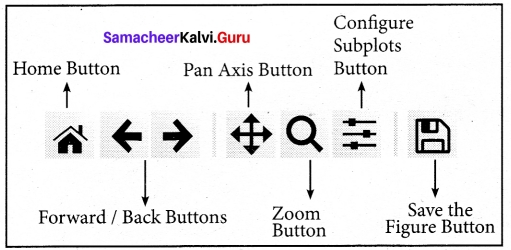Home Button → The Home Button will help once you have begun navigating your chart. If you ever want to return back to the original view, you can click on this.

Forward/Back buttons → These buttons can be used like the Forward and Back buttons in your browser. You can click these to move back to the previous point you were at, or forward again.

Pan Axis → This cross-looking button allows you to click it, and then click and drag your graph around.

Zoom → The Zoom button lets you click on it, then click and drag a square that you would like I to zoom into specifically. Zooming in will require a left click and drag. You can alternatively zoom out with a right click and drag.

Configure Subplots → This button allows you to configure various spacing options with your figure and plot.

Save Figure → This button will allow you to save your figure in various forms.

Question 37 (a).
Explain the purposes of
(a) pltxlabel
(b) plt.ylabel
(c) plt.title
(d) plt.legend()
(e) plt.show()
After installing Matplotlib, we will begin coding by importing Matplotlib using the command: import matplotlib.pyplot as pit
Now you have imported Matplotlib in your workspace. You need to display the plots. Using Matplotlib from within a Python script, you have to add plt.show() method inside the file to display your plot.

With plt.xlabel and plt.ylabel, you can assign labels to those respective axis. Next, you can assign the plot’s title with plt.title, and then you can invoke the default legend with pit.
legend().
plt.plot (years, total_populations)
plt.title (“Year vs Population in India”)
plt.xlabel (“Year”)
plt.ylabel (“Total Population”)
plt.legend()
plt.show()
Plt.title() → specifies title to the graph
Plt.xlabel() → specifies label for X-axis
Plt.ylabel() → specifies label for Y-axis[OR]

(b) Evaluate the following functions and write the output.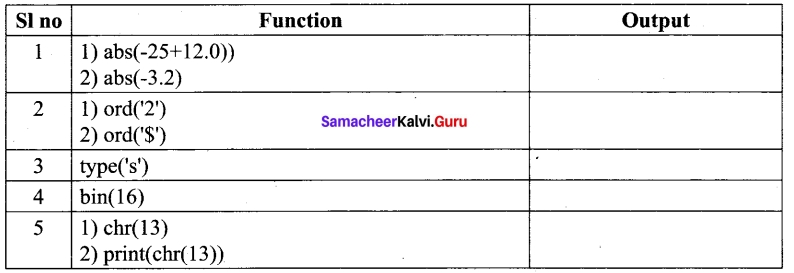Output:
1. 1) 13
2)3.2

2. (1)50
2) 36

3. <class ‘str’>

4. Ob10000

5. 1) CR (carriage return)
2) It moves the cursor to the beginning of same line

Question 38 (a).
Explain If and If…. else with sample programs.
Simple if statement
Simple if is the simplest of all decision making statements. Condition should be in the form of relational or logical expression.
Syntax:
if :
statements-block 1
In the above syntax if the condition is true statements – block 1 will be executed.
Example
# Program to check the age and print whether eligible for voting
x = int (input(“Enter your age :”))
if x> = 18:
print (“You are eligible for voting”)
Output 1:
You are eligible for voting Output 2:
>>>
As you can see in the second execution no output will be printed, only the Python prompt will be displayed because the program does not check the alternative process when the condition is failed.

if..else statement:
The if., else statement provides control to check the true block as well as the false block. Following is the syntax of ‘if.else’ statement.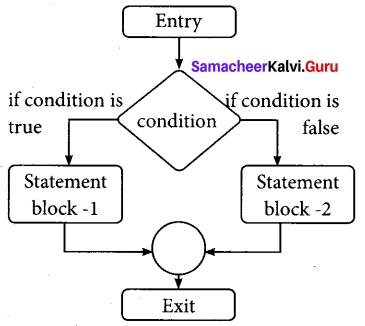Syntax:
if :
statements-block 1
else:
statements-block 2
if.else statement thus provides two possibilities and the condition determines which BLOCK is to be executed.
Example: #Program to check if the accepted number odd or even
a = int(input(“Enter any number :”))
if a%2 == 0:
print (a,” is an even number”)
else:
print (a, ” is an odd number”)
Output 1:
Enter any number :56
56 is an even number
Output 2:
Enter any number :67
67 is an odd number
An alternate method to rewrite the above program is also available in Python. The complete if.else can also written as:
Syntax:
variable = variable1 if condition else variable 2[OR]

(b) Explain while loop with sample program.
while loop
The syntax of while loop in Python has the following syntax: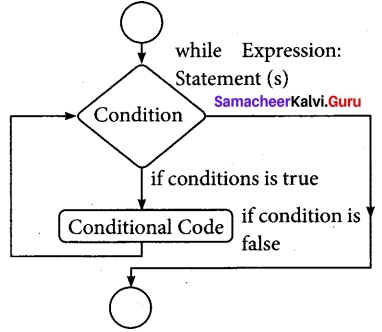Syntax:
while :
statements block 1
[else:
statements block 2]
In the while loop, the condition is any valid Boolean expression returning True or False. The else part of while is optional part of while. The statements blockl is kept executed till the condition is True. If the else part is written, it is executed when the condition is tested False. Recall while loop belongs to entry check loop type, that is it is not executed even once if the condition is tested False in the beginning.
Example: program to illustrate the use of while loop – to print all numbers from 10 to 15
i = 10 # initializing part of the control variable
while (i<= 15): # test condition
print (i,end=’\t’) # statements – block 1
i = i + l # Updation of the control variable
Output:
10 11 12 13 14 15